Thermodynamics and Propulsion

# 7.2 Microscopic and Macroscopic Descriptions of a System

The microscopic description of a system is the complete description of each particle in this system. In the above example, the microscopic description of the gas would be the list of the state of each molecule: position and velocity in this problem. It would require a great deal of data for this description; there are roughly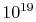molecules in a cube of air one centimeter on a side at room temperature and pressure. The macroscopic description, which is in terms of a few (two!) properties is thus far more accessible and useable for engineering applications, although it is restricted to equilibrium states.

To address the description of entropy on a microscopic level, we need to state some results concerning microscopic systems. These results and the computations and arguments below are taken almost entirely from the excellent discussion in Chapter 6 of Engineering Thermodynamics by Reynolds and Perkins7.1.

For a given macroscopic system, there are many microscopic states. A key idea from quantum mechanics is that the states of atoms, molecules, and entire systems are discretely quantized. This means that a system of particles under certain constraints, like being in a box of a specified size, or having a fixed total energy, can exist in a finite number of allowed microscopic states. This number can be very big, but it is finite. The microstates of the system keep changing with time from one quantum state to another as molecules move and collide with one another. The probability for the system to be in a particular quantum state is defined by its quantum-state probability. The set of theis called the distribution of probability. The sum of the probabilities of all the allowed quantum states must be unity, hence for any time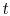,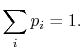(7..2)

When the system reaches equilibrium, the individual molecules still change from one quantum state to another. In equilibrium, however, the system state does not change with time; so the probabilities for the different quantum states are independent of time. This distribution is then called the equilibrium distribution, and the probabilitycan be viewed as the fraction of time a system spends in the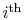quantum state. In what follows, we limit consideration to equilibrium states.

We can get back to macroscopic quantities from the microscopic description using the probability distribution. For instance, the macroscopic energy of the system would be the weighted average of the successive energies of the system (the energies of the quantum states); the energies are weighted by the relative time the system spends in the corresponding microstates. In terms of probabilities, the average energy,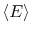, is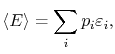(7..3)

where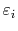is the energy of a quantum state.

The probability distribution provides information on the randomness of the equilibrium quantum states. For example, suppose the system can only exist in three states (1, 2 and 3). If the distribution probability is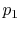the system is in quantum state 1 and there is no randomness. If we were asked what quantum state the system is in, we would be able to say it is always in state 1. If the distribution were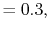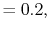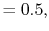orthe randomness would not be zero and would be equal in both cases. We would be more uncertain about the instantaneous quantum state than in the first situation.

Maximum randomness corresponds to the case where the three states are equally probable:In this case, we can only guess the instantaneous state with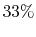probability.

UnifiedTP